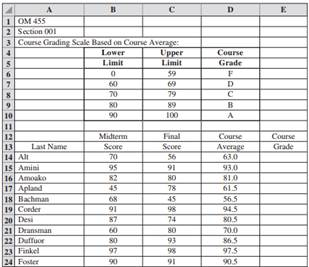### Create an Account

Home / Questions / Professor Rao would like to accurately calculate the grades for the 58 students in his Ope...

# Professor Rao would like to accurately calculate the grades for the 58 students in his Operations Planning and Scheduling class OM 455 He has thus far constructed a spreadsheet part of which

Professor Rao would like to accurately calculate the grades for the 58 students in his Operations Planning and Scheduling class (OM 455). He has thus far constructed a spreadsheet, part of which follows:a. The Course Average is calculated by weighting the Midterm Score and Final Score 50 percent each. Use the VLOOKUP function with the table shown to generate the Course Grade for each student in cells E14 through E24.

b. Use the COUNTIF function to determine the number of students receiving each letter grade.

Apr 25 2020 View more View LessSubscribe To Get Solution